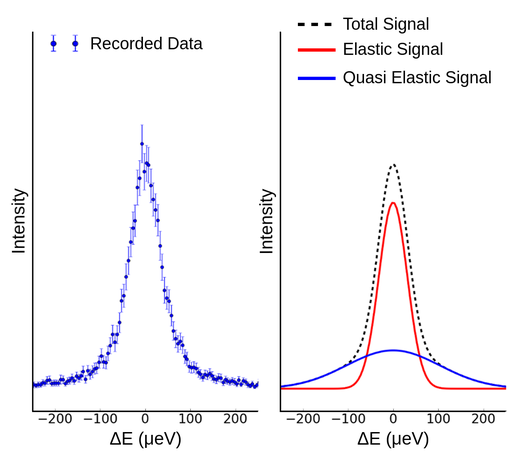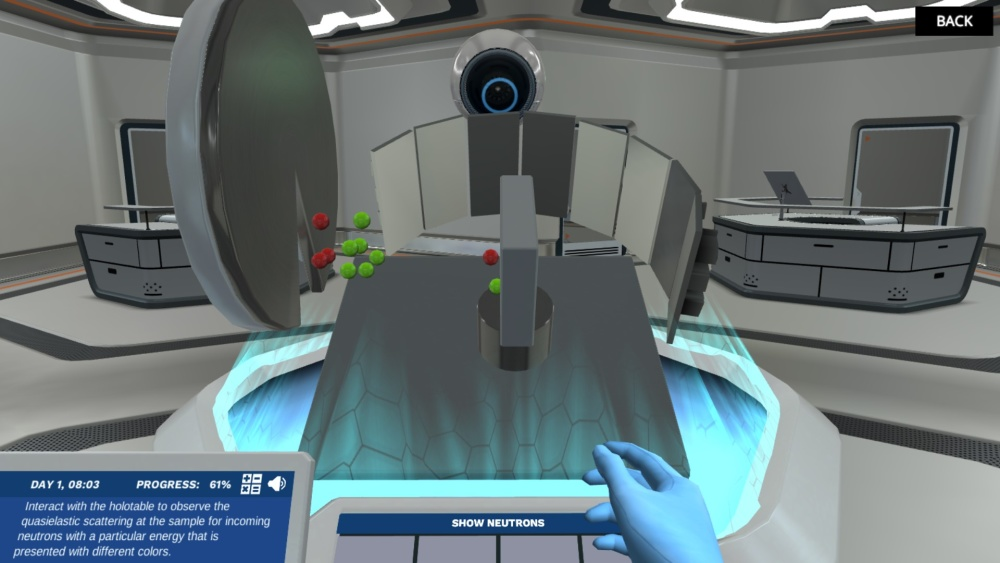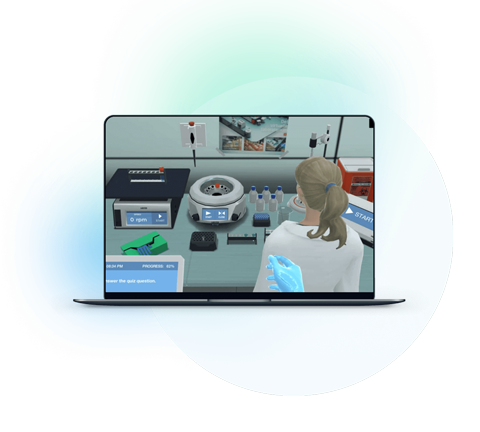Blog

# 5 Ways to Make Quasi-Elastic Neutron Scattering (QENS) Interesting for Students

Teaching with Labster
November 2, 2022

Quasi-elastic neutron scattering (QENS) is a technique that is used to measure dynamic processes in solid materials such as plastic, ceramics, gels, and many more. In QENS, we achieve information about atoms moving in the sample (via e.g. diffusion) through incoherent scattering. This is in contrast to diffraction, where we achieve information about the sample's atomic (static) structure through (elastic) coherent scattering.

Neutrons are subatomic particles and can be found in the nucleus of most atoms. The mass of a neutron is approximately the same as a proton mn = 1.675*10-27kg, but neutrons carry no electrical charge unlike protons. If neutrons are expelled from the nucleus, as is done purposefully at the spallation source facility, the neutrons will decay with a half time of τ = 886s – close to 15 minutes. This is well above the time it takes a neutron to travel from the source to the instrument in a neutron experiment, which is usually less than a second. Neutrons exist as both particles and waves, called the particle-wave duality, which is the cornerstone of quantum mechanics.

## Why do students face difficulties in learning quasi-elastic neutron scattering (QENS)?

The following are the three primary reasons that make materials science with neutrons challenging to understand.

### 1. Lack of background knowledge

Lack of basic knowledge increases the difficulties of understanding any topic. While dealing with nuclear chemistry, students must know about subatomic particles and their properties. Students must know about fusion and fission reactions. Students must know about the applications and safety measures of these reactions.

### 2. Combination of physics and chemistry

The major difficulty in nuclear science is that it involves both physics and chemistry. Students must know physics and chemistry to understand what is happening inside a neutron-containing cell. Teach the students about the chemistry and physics involved in nuclear reactions by making separate portions.

### 3. Theoretical imagination

Theoretical learning of this topic is complicated; we also cannot see these reactions practically. So the best option is video graphics. If you don't have the facility of multimedia, you can use your laptop enough; otherwise, draw the complete mechanism along with arrows identifying the reaction sites and products.

## 5 Ways to make quasi-elastic neutron scattering (QENS) exciting for students

### 1. Briefly explain the basics.

Following are few terms to know first before going to QENS:

Spallation: There are different ways of producing neutrons, but the one we will focus on here is spallation. Spallation occurs when a high power proton beam hits a heavy element target which releases neutrons.

Neutron Moderation: To slow down neutrons we use what is called a moderator. In the moderator the neutrons collide with atoms that roughly have the same mass as a neutron, such as hydrogen. In such a collision the loses half its energy on average and it thus takes about 25-30 collisions for the neutrons to cool from energies in the MeV (mega electron volt) range to the ten meV (milli electron volt) range.

Neutron Guides: Guides are used to transport the neutrons from the moderator to most of the instrument where the experiment is carried out. The guides are tubes made in a way to totally reflect as many neutrons as possible much like how an optical fiber would transport a beam of light.

Neutrons Choppers: For most neutron experiments it is not enough to simply have an intense neutron beam, we also need to have a well-defined beam. When talking about a well-defined beam, we mean that we often want to control the energy or wavelength of the neutrons, sometimes we want to control precisely the direction the neutrons are traveling in before they hit the sample, and we might want to control exactly where on the sample they hit. The first of these three goals can be achieved by either choppers or analyzers. The other two can be achieved by collimator and slits, respectively.

### 2. Explain quasi-elastic neutron scattering (QENS)

Quasi-elastic neutron scattering (QENS) is a technique which is used to measure dynamic processes in solid materials such as plastic, ceramics, gels and many more. In QENS we achieve information about atoms moving in the sample (via e.g. diffusion) through incoherent scattering. This is in contrast to diffraction where we achieve information about the atomic (static) structure of the sample through (elastic) coherent scattering. The name quasi-elastic refers to scattering events within materials where the energy of the neutron changes only slightly by delivering (or receiving) energy to/from the sample. If the energy change of the neutron is bigger, the process is called inelastic scattering. Sometimes the neutrons scatter from collective movements (as opposed to random movements) of atoms in the sample. This type of scattering is called coherent inelastic scattering, but we will not go in detail with that here.

The neutron energy losses (negative energy transfers) we observe in a QENS experiment occur because the neutrons push atoms in the sample which then start to move and gain momentum, but at the same time the neutron loses momentum and thus energy. On the other hand, neutrons can also gain momentum if pushed by atoms in the sample which we see as a positive energy transfer (neutron energy gain). This means we can measure the movement of atoms or molecules in a sample by looking at the change in energy of the neutrons.

In order to find the energy change of the neutrons during scattering at the sample we of course need to know the energy of the neutron before and after the sample scattering. You can learn more about how this is done in practice with monochromators, analysers and choppers by following the links. In the present QENS experiment the neutron energy after the sample is selected by an analyser to a fixed energy. The range of energies in the neutron beam before the sample is selected by a chopper-system. Some of those neutrons will be scattered quasi-elastically by the sample and thereby have or get the correct energy to pass the analyzer and finally reach the detector.

The chopper-system is also used to set the energy resolution of the instrument which is needed for each particular experiment. In general terms you need good energy resolution to observe slow movements in the sample whereas you can have a relatively poor energy resolution and still observe rapid movements of atoms in the sample.

### 3. Describe the polymer scattering and relaxation time

In a polymer structure like plastic or gels the individual polymer chain are intertwined into a mesh with an overall structure with ordering such as a favored orientation relative to each other, but it is not a rigid and precise structure as found in crystals. This means that the polymer chains can move inside the material. These movements are due to thermal fluctuations in the material and there are a number of different kinds of movement they can undergo. Bond rotation round the carbon backbone as shown in the figure, translations where the polymers slide through the net, and stretching where the polymers are stretched. Since these movements originate from thermal fluctuations, the directions are random, and the speed is in some way governed by the temperature. How much they can move depends on a number of factors, including the packing of the polymers, their weight, how much they are entangled and if they are attracted to each other by polar interaction.

Describing the polymers movement with a single number is complicated, we describe it through the relaxation time. The relaxation time refers to the speed at which the polymers reach equilibrium after an external force has been applied. What that means is the speed at which a deformation in the polymer mesh is removed by the movement of the polymer to fill any gaps so the material is in equilibrium. This speed or relaxation time is material dependent, imagine blowing a hole in olive oil or syrup. The hole in the olive oil would disappear faster than in the syrup. So in the example, syrup has a smaller relaxation time than oil since it takes a longer time for it to reach equilibrium. These two examples are liquids but the principle are the same for plastic, glass, living tissue and any other solid structures composed of long molecule chains.

Any object that moves through a polymer mesh will be slowed by the mesh dependent on the relaxation time of the polymers, since this relates to how fast the polymers can be “pushed to the side” by the object. This could be other polymers themselves or molecules that are bound to a polymer from the mesh “hitching a ride” through the mesh. The smaller the relaxation time, the larger the energy transfers (gain as well as loss) of the neutrons. This is generally seen in QENS experiments as a broadening of the peak. The relaxation time depends on the temperature of the material, in the data analysis of this simulation we have used the relation

(Iγ/π•(ΔE2+γ2))+background say> I per gamma divided by pi, multiplied by increase of E to the square, plus gamma to the square, plus background.

Where I is the intensity of the peak, ΔE is the energy change and γ is 1/relaxation time.

### 4. Graphical interpretation of QENS data

As in many other scattering techniques it is difficult or even impossible to get information directly from QENS data. A standard approach is to create a model, which is normally a mathematical expression of how the particles are moving, interacting or other hypothesis about the sample we want to investigate. From this model we can calculate what the QENS data should look like. This theoretical QENS data can then be compared to the experimental data. If the theoretical data and the experimental data matches, the model is a good description of system we are measuring. If the two data do not match, then the model is not a good description of the system. We can then make adjustment to the model, and check if that solved the problem so the model is a good description. We can keep making adjustments to the model until we have a good description of the system, or if we are unable to describe the experimental data using the model we have to make a new model and try again. This is called model fitting or model refinement and is perhaps one of the most fundamental parts of experimental scientific work. From the model, we can now learn something about our sample like the relaxation rate of the polymers in the sample. There are general observations that can be made without fitting a model if the system is simple enough. One simple relation is between the velocities if the particles in, for example, a polymer sample. The faster the particle moves the higher the possible energy transfers are.

The neutron instrument's energy resolution has to be considered when looking at QENS data. The resolution is the minimum energy change that the instrument can measure, so any change lower than the resolution we cannot distinguish. This means that the QENS peak will be wider in ΔE plot if the particles in the sample move faster, since we have a larger range of energy transfers happening.Figure 1. Example of QENS data (Image source Labster theory)

### 5. Use virtual lab simulations

With technological advances, it is much easier to explain complex and challenging processes with the help of simulations. Now, you can simulate experiments without the need for any valuable equipment.

In this regard, you can take help from Labster’s virtual lab simulations. These simulations engage students through interactive learning scenarios. Students dive into a 3D world, where they visually learn and apply their concepts to solving real-life problems.In Labster’s interactive Quasi-Elastic Neutron Scattering Virtual Lab, you will learn the basics of neutrons and how they can be used as probes in materials science. You will get to perform experiments in a modern large-scale research facility that requires special permission to enter.What exactly are virtual labs?